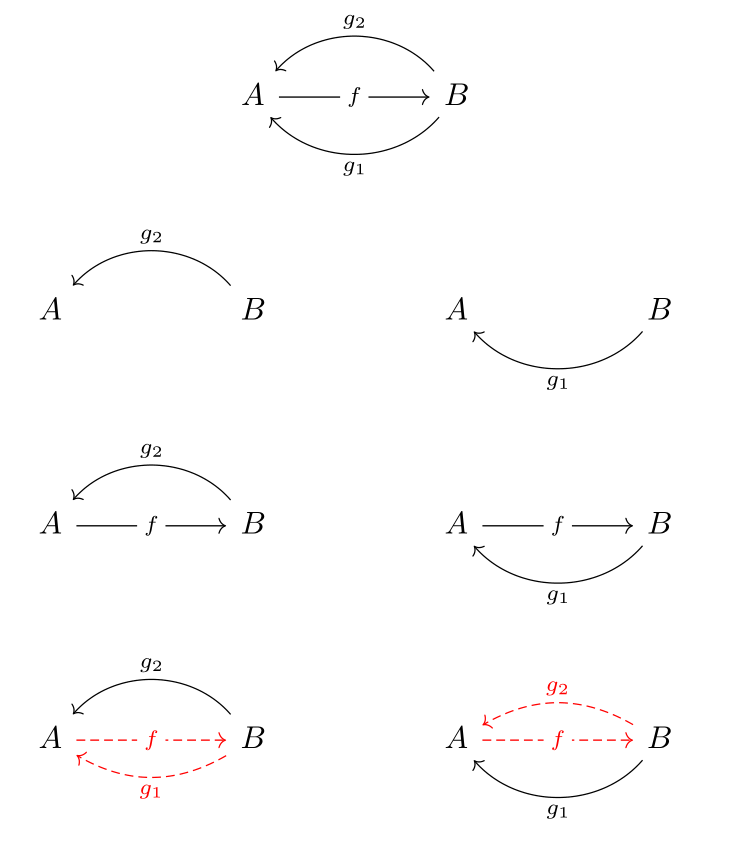Show Question
Math and science::Algebra::Aluffi

# Isomorphism

For functions between sets, we defined the notion of injective, surjective and bijective/isomorphic functions. We do the same for morphisms between objects of a category. This card covers isomorphisms; other cards cover monomorphisms and epimorphisms.

An isomorphism is defined as follows:

### Isomorphism. Definition.

Let $$C$$ be a category. A morphism $$f \in \cathom{C}(A, B)$$ is an isomorphism iff there exists a morphism $$g \in \cathom{C}(B, A)$$ such that both:

$gf = 1_A, \quad fg = 1_B.$

$$g$$ is said to be a (two sided) inverse of $$f$$.

### Uniqueness

Can $$f$$ have multiple inverses? Uniqueness of $$g$$ is not built into the definition above; however, it is indeed true that $$g$$ is unique:

### Theorem

An inverse of an isomorphism is unique.

Proof on the other side.

Since an inverse is unique, there is no ambiguity in denoting it as $$f^{-1}$$.

#### Auxiliary propositions

Here are three useful propositions related to isomorphisms:

• Every identity is an isomorphism and is its own inverse.
• If $$f$$ is an isomorphism then $$f^{-1}$$ is an isomorphism and $$(f^{-1})^{-1} = f$$.
• If $$f \in \cathom{C}(A, B)$$ and $$g \in \cathom{C}(B, C)$$ are isomorphisms, then $$g\, f$$ is an isomorphism and $$(g \, f)^{-1} = f^{-1}g^{-1}$$.

### An inverse of an isomorphism is unique. Proof.

Let $$f : A \to B$$ be a morphism, let $$g_1 : B \to A$$ be a left-inverse of $$f$$ and let $$g_2 : B \to A$$ be a right-inverse of $$f$$. Thus,

$g_1 \,f = 1_A, \quad f \, g_2 = 1_B$

By composing $$g_1$$, $$f$$ and $$g_2$$ then applying associativity we see that $$g_1 = g_2$$ and that this morphism is the unique inverse:

$g_1 (f \, g_2) = g_1 \, 1_B = g_1, \quad (g_1 \, f) g_2 = 1_A \, g_2 = g_2$

The fact that $$g_1 ( f \, g_2) = (g_1 \, f) g_2$$ is asserted by the definition of categories. Applying this we arrive at $$g_1 = g_2$$.

#### Intuition

The diagram below might spark some intuition. The composition of $$g_1$$, $$f$$ and $$g_2$$ is presented in the two possible: the left side constructs the path beginning with $$g_2$$ and the right side beginning with $$g_1$$.One intuitively appealing description of the diagram is that there is a three step journey where the middle leg, $$f$$, can negate either the first leg, $$g_2$$, or the last leg $$g_1$$. Regardless of which one is negated, the overall journey is the same (associativity). If $$g_1$$ is negated, then the journey is $$g_2$$, and if $$g_2$$ is negated the journey is $$g_1$$, but either way, the journel is the same, so $$g_1 = g_2$$.

### Departure from $$\mathrm{Set}$$

While many aspects of functions on sets transfer nicely to the catagorical setting, it is noteworthy that a morphism that is monomorphic and epimorphic (concepts which are analogous to injectivity and surjectivity of functions) may not necessarily be isomorphic. In comparison, a function is both injective and surjective iff it is bijective.

p28